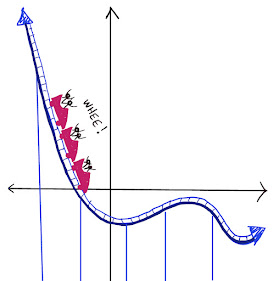## Monday, February 2, 2015

### Stacked Dice

Solution: The sum of any two opposite faces of a die is 7.  So, excluding the top face, the sum of the numbers on the 3-dice tower is always equal to 7 x 2 x 3 = 42, and it doesn't matter how we arrange the dice.  The only thing that affects the total sum is the number showing on the top, which can be any number from 1 to 6.  So the minimum sum is 42 + 1 = 43 and the maximum sum is 42 + 6 = 48.  For 20 dice, the minimum sum is 7 x 2 x 20 + 1 = 281 and the the maximum sum is 7 x 2 x 20 + 6 = 286.  For n dice, the minimum sum is 7 x 2 x n + 1 and the maximum sum is 7 x 2 x n + 6.

### PLAYFUL MATH BLOG CARNIVAL #163

BLOG CARNIVAL #163....LET'S GO! Fun fact: The number 163 is prime, which we can prove simply by showing that it is not divisible by 2, 3...